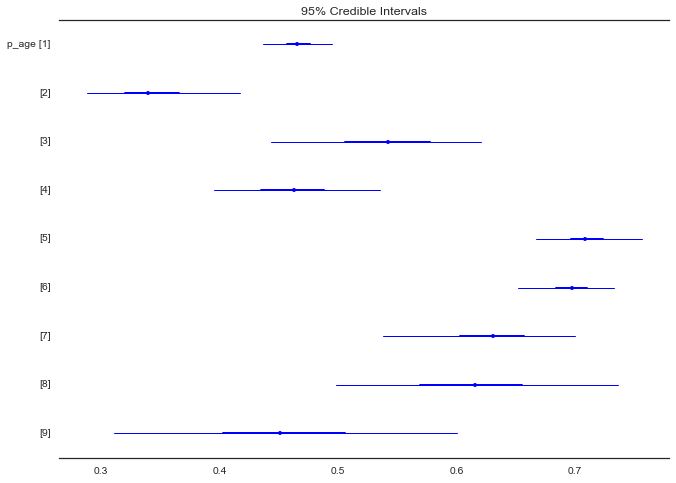# Disease Outbreak Response Decision-making Under Uncertainty: A retrospective analysis of measles in Sao Paulo¶

In :
%matplotlib inline
import pandas as pd
import numpy as np
import numpy.ma as ma
from datetime import datetime
import matplotlib.pyplot as plt
from pymc import MCMC, Matplot
from pymc import (Uniform, DiscreteUniform, Beta, Lambda, Binomial, Normal,
Poisson, NegativeBinomial, observed, negative_binomial_like, poisson_like,
Lognormal, Exponential, binomial_like, stochastic, potential,
invlogit, TruncatedNormal, Binomial, Gamma, HalfCauchy, normal_like,
deterministic, MvNormalCov, Bernoulli, potential, Uninformative,
Multinomial, rmultinomial, rbinomial, AdaptiveMetropolis,
Dirichlet, multinomial_like)

import ipdb

from IPython.core.display import HTML
def css_styling():
styles = open("styles/custom.css", "r").read()
return HTML(styles)
css_styling()

Out:
In :
data_dir = "data/"


Import outbreak data

In :
measles_data = pd.read_csv(data_dir+"measles.csv", index_col=0)
measles_data.BIRTH = pd.to_datetime(measles_data.BIRTH)
measles_data.ONSET = pd.to_datetime(measles_data.ONSET)

In :
measles_data = measles_data.replace({'DISTRICT': {'BRASILANDIA':'BRAZILANDIA'}})


Sao Paulo population by district

In :
sp_pop = pd.read_csv(data_dir+'sp_pop.csv', index_col=0)

In :
_names = sp_pop.index.values
_names[_names=='BRASILANDIA'] = 'BRAZILANDIA'
sp_pop.set_index(_names, inplace = True)

In :
sp_pop.head()

Out:
0 a 4 anos 5 a 9 anos 10 a 14 anos 15 a 19 anos 20 a 24 anos 25 a 29 anos 30 a 34 anos 35 a 39 anos 40 a 44 anos 45 a 49 anos 50 a 54 anos 55 a 59 anos 60 a 64 anos 65 a 69 anos 70 a 74 anos 75 anos e + Total
AGUA RASA 5411 5750 6450 7122 7621 7340 6999 6984 6346 5608 4987 4212 4152 3595 2937 3637 89151
ALTO DE PINHEIROS 2070 2369 2953 3661 4612 4190 3539 3633 3448 3289 3040 2533 2298 1732 1305 1823 46495
ANHANGUERA 3068 3006 2755 2431 2426 2636 2695 2308 1653 1107 753 509 352 217 162 171 26249
ARICANDUVA 7732 7730 8373 8956 9182 8531 7813 7365 6551 5554 4887 3858 3320 2449 1611 1723 95635
ARTUR ALVIM 9031 9078 10000 11058 11387 10347 9125 8658 7830 7055 5919 4612 3756 2633 1727 1724 113940

Plot of cumulative cases by district

In :
measles_onset_dist = measles_data.groupby(['DISTRICT','ONSET']).size().unstack(level=0).fillna(0)
measles_onset_dist.cumsum().plot(legend=False, grid=False)

Out:
<matplotlib.axes._subplots.AxesSubplot at 0x10be6f908>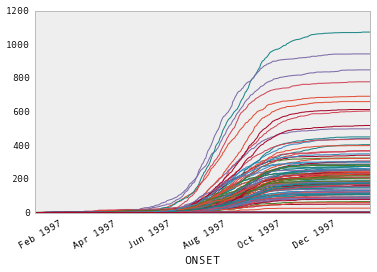In :
total_district_cases = measles_onset_dist.sum()


Top 5 districts by number of cases

In :
totals = measles_onset_dist.sum()
totals.sort(ascending=False)
totals[:5]

Out:
DISTRICT
GRAJAU             1074
JARDIM ANGELA       944
CAPAO REDONDO       849
JARDIM SAO LUIZ     778
CAMPO LIMPO         692
dtype: float64

Age distribution of cases, by confirmation status

In :
by_conclusion = measles_data.groupby(["YEAR_AGE", "CONCLUSION"])
counts_by_cause = by_conclusion.size().unstack().fillna(0)
ax = counts_by_cause.plot(kind='bar', stacked=True, xlim=(0,50), figsize=(15,5))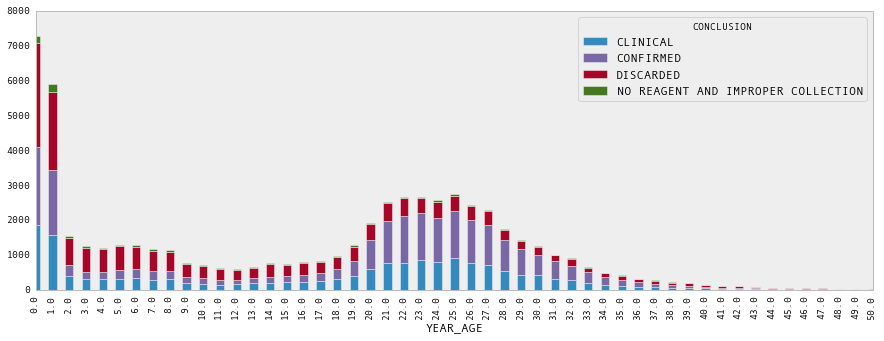## Stochastic Disease Transmission Model¶

As a baseline for comparison, we can fit a model to all the clinically-confirmed cases, regardless of lab confirmation status. For this, we will use a simple SIR disease model, which will be fit using MCMC.

This model fits the series of 2-week infection totals in each district $i$ as a set of Poisson models:

$$Pr(I(t)_{i} | \lambda(t)_i) = \text{Poisson}(\lambda(t)_i)$$

Where the outbreak intensity is modeled as:

$$\lambda(t)_i = \beta [I^{(w)}(t-1)_i]^{\alpha} S(t-1)_i$$

$$\alpha \sim \text{Exp}(1)$$

We will assume here that the transmission rate is constant over time (and across districts):

$$\beta \sim \text{Gamma}(1, 0.1)$$

To account for the influence of infected individuals from neighboring districts on new infections, the outbreak intensity was modeled using a spatial-weighted average of infecteds across districts, where populations were weighted as an exponential function of the distance between district centroids:

$$w_{d} = \text{exp}(-\theta d)$$

$$\theta \sim \text{Exp}(1)$$

### Confirmation Sub-model¶

Rather than assume all clinical cases are true cases, we can adjust the model to account for lab confirmation probability. This is done by including a sub-model that estimates age group-specific probabilities of confirmation, and using these probabilities to estimate the number of lab-confirmed cases. These estimates are then plugged into the model in place of the clinically-confirmed cases.

We specified a structured confirmation model to retrospectively determine the age group-specific probabilities of lab confirmation for measles, conditional on clinical diagnosis. Individual lab confirmation events $c_i$ were modeled as Bernoulli random variables, with the probability of confirmation being allowed to vary by age group:

$$c_i \sim \text{Bernoulli}(p_{a(i)})$$

where $a(i)$ denotes the appropriate age group for the individual indexed by i. There were 16 age groups, the first 15 of which were 5-year age intervals $[0,5), [5, 10), \ldots , [70, 75)$, with the 16th interval including all individuals 75 years and older.

Since the age interval choices were arbitrary, and the confirmation probabilities of adjacent groups likely correlated, we modeled the correlation structure directly, using a multivariate logit-normal model. Specifically, we allowed first-order autocorrelation among the age groups, whereby the variance-covariance matrix retained a tridiagonal structure.

\begin{aligned} \Sigma = \left[{ \begin{array}{c} {\sigma^2} & {\sigma^2 \rho} & 0& \ldots & {0} & {0} \\ {\sigma^2 \rho} & {\sigma^2} & \sigma^2 \rho & \ldots & {0} & {0} \\ {0} & \sigma^2 \rho & {\sigma^2} & \ldots & {0} & {0} \\ \vdots & \vdots & \vdots & & \vdots & \vdots\\ {0} & {0} & 0 & \ldots & {\sigma^2} & \sigma^2 \rho \\ {0} & {0} & 0 & \ldots & \sigma^2 \rho & {\sigma^2} \end{array} }\right] \end{aligned}

From this, the confirmation probabilities were specified as multivariate normal on the inverse-logit scale.

$$\text{logit}(p_a) = \{a\} \sim N(\mu, \Sigma)$$

Priors for the confirmation sub-model were specified by:

\begin{aligned} \mu_i &\sim N(0, 100) \\ \sigma &\sim \text{HalfCauchy}(25) \\ \rho &\sim U(-1, 1) \end{aligned}

Age classes are defined in 5-year intervals.

In :
age_classes = [0,5,10,15,20,25,30,35,40,100]
measles_data.dropna(subset=['YEAR_AGE'], inplace=True)
measles_data['YEAR_AGE'] = measles_data.YEAR_AGE.astype(int)
measles_data['AGE_GROUP'] = pd.cut(measles_data.AGE, age_classes, right=False)


Lab-checked observations are extracted for use in estimating lab confirmation probability.

In :
CONFIRMED = measles_data.CONCLUSION == 'CONFIRMED'
CLINICAL = measles_data.CONCLUSION == 'CLINICAL'


Extract confirmed and clinical subset, with no missing county information.

In :
lab_subset = measles_data[(CONFIRMED | CLINICAL) & measles_data.COUNTY.notnull()].copy()

In :
age = lab_subset.YEAR_AGE.values
ages = lab_subset.YEAR_AGE.unique()
counties = lab_subset.COUNTY.unique()
y = (lab_subset.CONCLUSION=='CONFIRMED').values

In :
_lab_subset = lab_subset.replace({"CONCLUSION": {"CLINICAL": "UNCONFIRMED"}})
by_conclusion = _lab_subset.groupby(["YEAR_AGE", "CONCLUSION"])
counts_by_cause = by_conclusion.size().unstack().fillna(0)
ax = counts_by_cause.plot(kind='bar', stacked=True, xlim=(-1,50), figsize=(15,5), grid=False)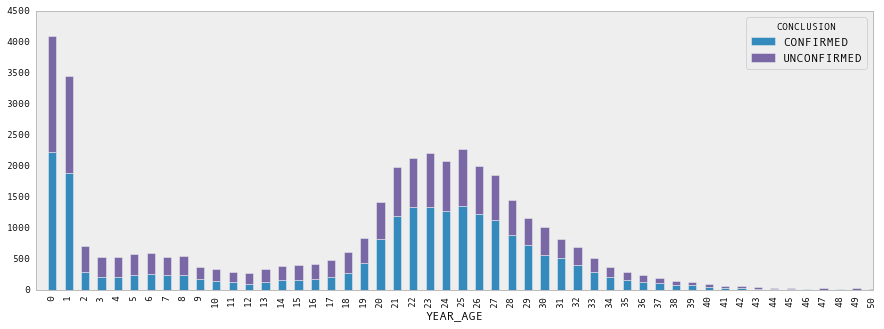In :
lab_subset.shape

Out:
(39982, 16)
In :
y.sum()

Out:
22097

Proportion of lab-confirmed cases older than 20 years

In :
(measles_data[CONFIRMED].YEAR_AGE>20).mean()

Out:
0.60257048468117846
In :
# Get index from full crosstabulation to use as index for each district
dates_index = measles_data.groupby(
['ONSET', 'AGE_GROUP']).size().unstack().index

In :
unique_districts = measles_data.DISTRICT.dropna().unique()

In :
excludes = ['BOM RETIRO']

In :
N = sp_pop.ix[unique_districts, 'Total'].dropna()
N = N.drop(excludes)

In :
sp_districts = N.index.values
len(sp_districts)

Out:
92

Compile bi-weekly confirmed and unconfirmed data by Sao Paulo district

In :
all_district_data = []
all_confirmed_cases = []
for d in sp_districts:

# All bi-weekly unconfirmed and confirmed cases
district_data = lab_subset[lab_subset.DISTRICT==d]
district_counts_2w = district_data.groupby(
['ONSET', 'AGE_GROUP']).size().unstack().reindex(dates_index).fillna(0).resample('2W', how='sum')
all_district_data.append(district_counts_2w)

# All confirmed cases, by district
confirmed_data = district_data[district_data.CONCLUSION=='CONFIRMED']
confirmed_counts = confirmed_data.groupby(
['ONSET', 'AGE_GROUP']).size().unstack().reindex(dates_index).fillna(0).sum()
all_confirmed_cases.append(confirmed_counts.reindex_axis(measles_data['AGE_GROUP'].unique()).fillna(0))


Time series of cases by district, summarized in 2-week intervals

In :
# Sum over ages for susceptibles
sp_cases_2w = [dist.sum(1) for dist in all_district_data]
len(sp_cases_2w)

Out:
92
In :
measles_data.AGE_GROUP.cat.categories

Out:
Index(['[0, 5)', '[5, 10)', '[10, 15)', '[15, 20)', '[20, 25)', '[25, 30)',
'[30, 35)', '[35, 40)', '[40, 100)'],
dtype='object')
In :
# Ensure the age groups are ordered
I_obs = np.array([dist.reindex_axis(measles_data.AGE_GROUP.cat.categories,
axis=1).fillna(0).values.astype(int) for dist in all_district_data])

In :
# Sum over districts
I_obs = I_obs.sum(0)

In :
I_obs.max()

Out:
641
In :
I_obs.sum()

Out:
16640
In :
age_groups = measles_data.AGE_GROUP.cat.categories
age_groups

Out:
Index(['[0, 5)', '[5, 10)', '[10, 15)', '[15, 20)', '[20, 25)', '[25, 30)',
'[30, 35)', '[35, 40)', '[40, 100)'],
dtype='object')

Check shape of data frame

• 28 bi-monthly intervals, 9 age groups
In :
assert I_obs.shape == (28, len(age_groups))


Prior distribution on susceptible proportion:

$$p_s \sim \text{Beta}(2, 100)$$

In :
from pymc import rbeta
plt.hist(rbeta(2, 100, 10000))

Out:
(array([  3.16300000e+03,   3.52400000e+03,   1.88500000e+03,
8.77000000e+02,   3.65000000e+02,   1.19000000e+02,
3.50000000e+01,   2.30000000e+01,   6.00000000e+00,
3.00000000e+00]),
array([ 0.00020843,  0.01143471,  0.02266099,  0.03388727,  0.04511355,
0.05633983,  0.06756612,  0.0787924 ,  0.09001868,  0.10124496,
0.11247124]),
<a list of 10 Patch objects>)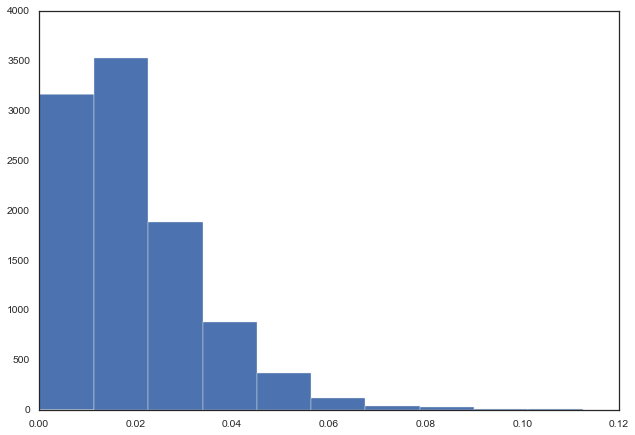In :
obs_date = '1997-06-15'
obs_index = all_district_data.index <= obs_date
I_obs_t = I_obs[obs_index]

In :
#Extract cases by age and time.

age_group = pd.cut(age, age_classes, right=False)
#age_index = np.array([age_group.categories.tolist().index(i) for i in age_group])
age_index = pd.cut(lab_subset.AGE[lab_subset.ONSET < obs_date], age_classes, right=False, labels=False)

In :
confirmed = (lab_subset[lab_subset.ONSET < obs_date].CONCLUSION=='CONFIRMED').values


Empirical confirmation

In :
ax = pd.DataFrame({'age':age_index,
'confirmed':confirmed}).groupby('age')['confirmed'].mean().plot(kind='bar')
ax.set_xticklabels(age_group.categories);
ax.set_ylabel('Confirmation')

Out:
<matplotlib.text.Text at 0x1206cdb70>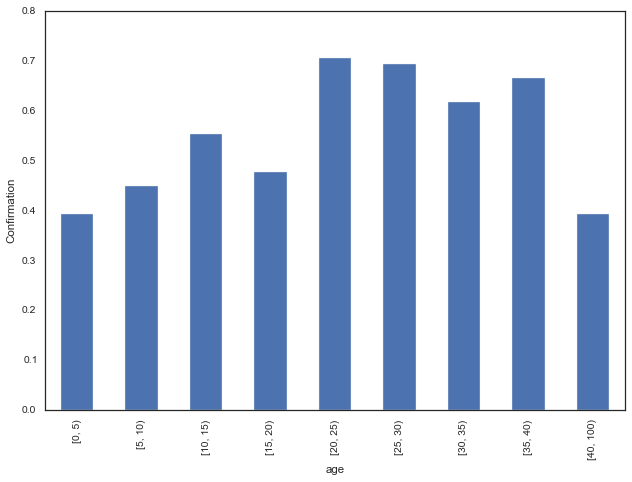In :
np.sum(I_obs_t, (0)) / float(I_obs_t.sum())

Out:
array([ 0.11950395,  0.18038331,  0.06989853,  0.06087937,  0.24802706,
0.21984216,  0.06989853,  0.02029312,  0.01127396])
In :
I_obs_t

Out:
array([[  0,   1,   0,   0,   0,   0,   0,   0,   0],
[  3,   2,   1,   0,   0,   2,   0,   0,   0],
[  1,   5,   0,   0,   1,   0,   0,   0,   0],
[  4,   2,   0,   0,   1,   1,   0,   0,   0],
[  3,   1,   2,   4,   4,   1,   1,   0,   1],
[  6,   5,   2,   4,   8,  10,   4,   0,   0],
[  5,  14,   2,   5,   6,   2,   1,   0,   0],
[  8,  10,   2,   3,   6,   5,   1,   1,   1],
[  6,   9,   3,   4,  13,   6,   5,   0,   1],
[ 13,  21,   8,   8,  23,  26,   9,   5,   0],
[ 19,  38,  12,   9,  64,  41,  14,   4,   5],
[ 38,  52,  30,  17,  94, 101,  27,   8,   2]])
In :
I_obs_t.sum((0,1))

Out:
887
In :
def measles_model(obs_date, confirmation=True, spatial_weighting=False, all_traces=False):

'''
Truncate data at observation period
'''
obs_index = all_district_data.index <= obs_date
I_obs_t = I_obs[obs_index]

age = lab_subset[lab_subset.ONSET < obs_date].YEAR_AGE.values
age_cutpoints = [0,5,10,15,20,25,30,35,40,100]
#     age_group = pd.cut(age, age_cutpoints, right=False)
age_index = pd.cut(age, age_cutpoints, right=False, labels=False)
# Indicators for lab-checked individuals who were confirmed
confirmed = (lab_subset[lab_subset.ONSET < obs_date].CONCLUSION=='CONFIRMED').values

# Index for observation date, used to index out values of interest
# from the model.
t_obs = obs_index.sum() - 1

n_periods, n_age_groups = I_obs_t.shape

### Confirmation sub-model

if confirmation:

# Specify priors on age-specific means
age_classes = np.unique(age_index)

mu = Normal("mu", mu=0, tau=0.0001, value=*len(age_classes))
sig = HalfCauchy('sig', 0, 25, value=1)
tau = sig**-2

# Age-specific probabilities of confirmation as multivariate normal
# random variables
beta_age = Normal("beta_age", mu=mu, tau=tau,
value=*len(age_classes))
p_age = Lambda('p_age', lambda t=beta_age: invlogit(t))

@deterministic()
def p_confirm(beta=beta_age):
return invlogit(beta[age_index])

# Confirmation likelihood
lab_confirmed = Bernoulli('lab_confirmed', p=p_confirm, value=confirmed,
observed=True)

@stochastic(trace=all_traces, dtype=int)
def I(value=(I_obs_t).astype(int), n=I_obs_t, p=p_age):
# Binomial confirmation process: confirm by age, then re-combine

return np.sum([binomial_like(value[:,a], n[:,a], p[a])
for a in range(n_age_groups)])

age_dist_init = np.sum(I.value, 0)/ float(I.value.sum())

else:

I = I_obs_t
age_dist_init = np.sum(I, 0) / float(I.sum())

assert I.shape == (t_obs +1, n_age_groups)

# Calcuate age distribution from observed distribution of infecteds to date
age_dist = Dirichlet('age_dist', np.ones(n_age_groups), value=age_dist_init[:-1]/age_dist_init.sum())
@potential
def age_dist_like(p=age_dist, I=I):
p = np.append(p, 1-p.sum())
return multinomial_like(I.sum(0), I.sum(), p)

# Weakly-informative prior on proportion susceptible being
# between 0 and 0.07
p_susceptible = 0.04 #Beta('p_susceptible', 2, 100, value=0.06)

# Estimated total initial susceptibles
S_0 = Binomial('S_0', n=int(N.sum()), p=p_susceptible)

# Calculate susceptibles from total number of infections
S = Lambda('S', lambda I=I, S_0=S_0: S_0 - I.sum(1).cumsum())

# Check shape
assert S.value.shape == t_obs+1

# Susceptibles at time t, by age
@deterministic
def S_age(S=S, p=age_dist):
p = np.append(p, 1-p.sum())
return rmultinomial(S[-1], p)

assert S_age.value.shape == (n_age_groups,)

# Transmission parameter
β = Uniform('β', 1, 50, value=10)

α = 1 #= Exponential('α', 1, value=1)

# Force of infection
@deterministic
def λ(β=β, I=I, S=S, α=α):

return β * I.sum(1)**α * S / N.sum()

# Check shape
assert λ.value.shape == (t_obs+1,)

# FOI in observation period
λ_t = Lambda('λ_t', lambda λ=λ: λ[-1])

# Poisson likelihood for observed cases
@potential
def new_cases(I=I, λ=λ):
#         x = I.sum(1)
#         return negative_binomial_like(x[1:], λ[:-1], x[:-1]+1)
return poisson_like(I.sum(1)[1:], λ[:-1])

'''
Vaccination targets
'''

@deterministic
def vacc_5(S=S_age):
# Vaccination of 15 and under
p = [0.95] + *(n_age_groups-1)
return rbinomial(S, p)

# Proportion of susceptibles vaccinated
pct_5 = Lambda('pct_5',
lambda V=vacc_5, S=S_age: float(V.sum())/S.sum())

@deterministic
def vacc_15(S=S_age):
# Vaccination of 15 and under
p = [0.95]*3 + *(n_age_groups-3)
return rbinomial(S, p)

# Proportion of susceptibles vaccinated
pct_15 = Lambda('pct_15',
lambda V=vacc_15, S=S_age: float(V.sum())/S.sum())

@deterministic
def vacc_30(S=S_age):
# Vaccination of 30 and under
p = [0.95]*6 + *(n_age_groups-6)
return rbinomial(S, p)

# Proportion of 30 and under susceptibles vaccinated
pct_30 = Lambda('pct_30',
lambda V=vacc_30, S=S_age: float(V.sum())/S.sum())

@deterministic
# Vaccination of adults under 30 (and young kids)
p = [0.95, 0, 0, 0, 0.95, 0.95] + *(n_age_groups-6)
return rbinomial(S, p)

# Proportion of adults under 30 (and young kids)
lambda V=vacc_adult, S=S_age: float(V.sum())/S.sum())

return locals()


Run models for June 15 and July 15 observation points, both with and without clinical confirmation.

In :
db = 'ram'
n_iterations = 50000
n_burn = 40000


June 15, with lab confirmation

In :
model = measles_model

In :
from pymc import Slicer

model_june = MCMC(model('1997-06-15'), db=db, dbname='model_june')

In :
model_june.stochastics

Out:
{<pymc.distributions.new_dist_class.<locals>.new_class 'sig' at 0x120de9e10>,
<pymc.distributions.new_dist_class.<locals>.new_class 'β' at 0x10e4aac18>,
<pymc.distributions.new_dist_class.<locals>.new_class 'mu' at 0x120de9c50>,
<pymc.distributions.new_dist_class.<locals>.new_class 'age_dist' at 0x10c609668>,
<pymc.distributions.new_dist_class.<locals>.new_class 'S_0' at 0x120ce9908>,
<pymc.PyMCObjects.Stochastic 'I' at 0x10c612d68>,
<pymc.distributions.new_dist_class.<locals>.new_class 'beta_age' at 0x120de9f60>}
In :
model_june.sample(n_iterations, n_burn)

 [-----------------100%-----------------] 50000 of 50000 complete in 56.2 sec

### July 15, with lab confirmation¶

In :
model_july = MCMC(model('1997-07-15'), db=db, dbname='model_july')
# model_july.use_step_method(AdaptiveMetropolis, [model_july.β, model_july.p_susceptible])

In :
model_july.sample(n_iterations, n_burn)

 [-----------------100%-----------------] 50000 of 50000 complete in 66.3 sec

June 15, no lab confirmation

In :
model_june_noconf = MCMC(model('1997-06-15', confirmation=False),
db=db, dbname='model_june_noconf')
# model_june_noconf.use_step_method(Slicer, model_june_noconf.beta_age)
# model_june_noconf.use_step_method(Slicer, model_june_noconf.p_susceptible)

In :
model_june_noconf.sample(n_iterations, n_burn)

 [-----------------100%-----------------] 50000 of 50000 complete in 24.4 sec

July 15, no lab confirmation

In :
model_july_noconf = MCMC(model('1997-07-15',
confirmation=False),
db=db, dbname='model_july_noconf')
# model_july_noconf.use_step_method(AdaptiveMetropolis, [model_july_noconf.β, model_july_noconf.p_susceptible])

In :
model_july_noconf.sample(n_iterations, n_burn)

 [-----------------100%-----------------] 50000 of 50000 complete in 23.7 sec

## Summary of model output¶

Distance weighting parameter for june model with confirmation

In :
Matplot.plot(model_june.S_0)

Plotting S_0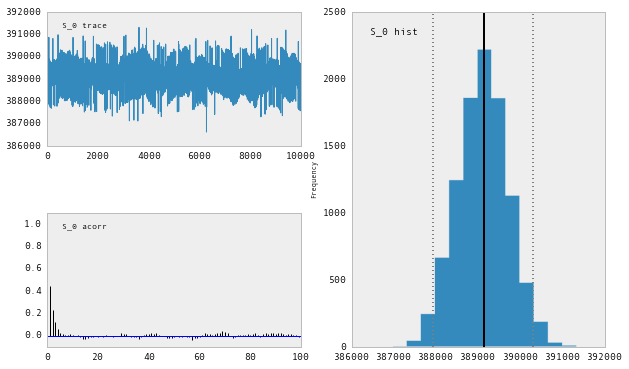Lab confirmation rates, June model

In :
import seaborn as sb

p_age = pd.DataFrame(model_june.p_age.trace(), columns=age_group.categories)

f, axes = plt.subplots(figsize=(14,6))
sb.boxplot(data=p_age, linewidth=0.3, fliersize=0, ax=axes,
color=sb.color_palette("coolwarm", 5),
order=age_group.categories)
axes.set_ylabel('Confirmation rate')
axes.set_xlabel('Age group')
axes.set_title('July confirmation estimates')

Out:
<matplotlib.text.Text at 0x10b733f98>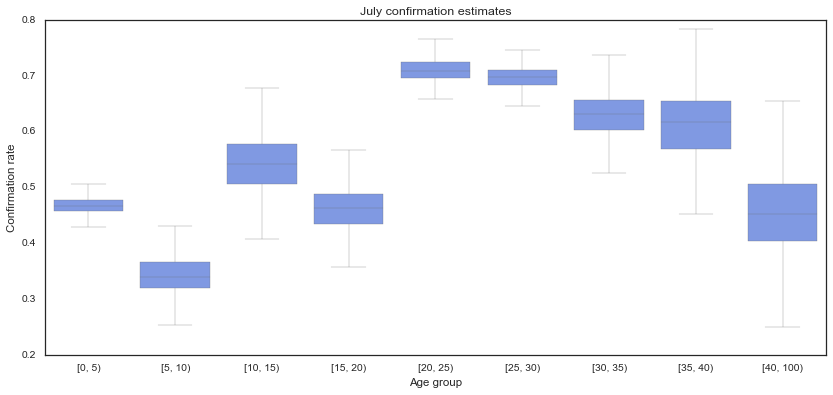In :
import seaborn as sb

p_age = pd.DataFrame(model_july.p_age.trace(), columns=age_group.categories)

f, axes = plt.subplots(figsize=(14,6))
sb.boxplot(data=p_age, linewidth=0.3, fliersize=0, ax=axes,
color=sb.color_palette("coolwarm", 5),
order=age_group.categories)
axes.set_ylabel('Confirmation rate')
axes.set_xlabel('Age group')
axes.set_title('June confirmation estimates')

Out:
<matplotlib.text.Text at 0x1185ca470>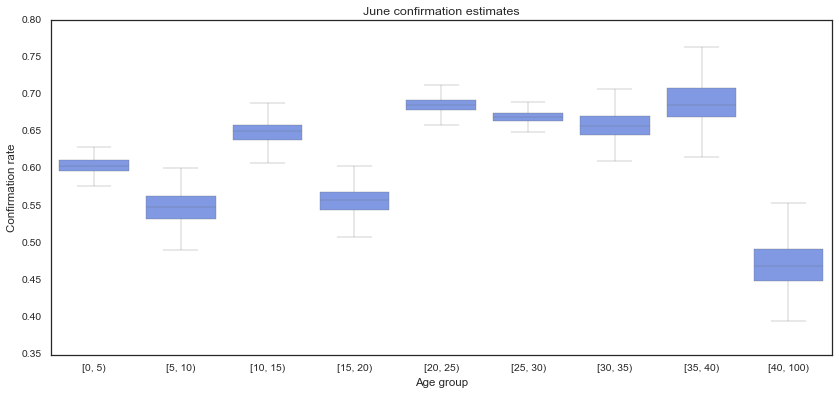Proportion of population susceptible, June model.

In :
Matplot.plot(model_june.β)

Plotting β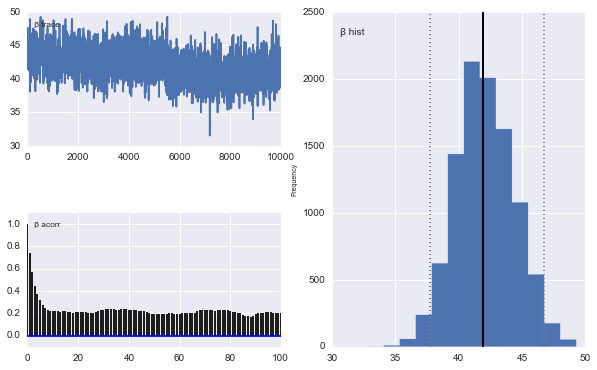Proportion of population susceptible, June model with no confirmation correction

In :
Matplot.plot(model_june.p_susceptible)

---------------------------------------------------------------------------
TypeError                                 Traceback (most recent call last)
<ipython-input-70-f73097280a32> in <module>()
----> 1 Matplot.plot(model_june.p_susceptible)

/Users/fonnescj/GitHub/pymc/pymc/Matplot.py in wrapper(pymc_obj, *args, **kwargs)
375
376         # If others fail, assume that raw data is passed
--> 377         f(pymc_obj, *args, **kwargs)
378
379     wrapper.__doc__ = f.__doc__

TypeError: plot() missing 1 required positional argument: 'name'

Epidemic intensity estimates at June and July, per district.

In :
Matplot.plot(model_june.λ_t)

Plotting λ_t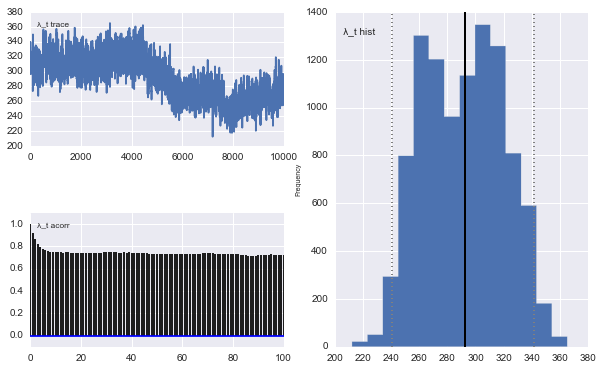Epidemic intensity for lab- versus clinical-confirmation models

In :
lam_june = model_june.λ.stats()

fig, axes = plt.subplots(2, 1, sharey=True)

axes.plot(lam_june['quantiles'].T, 'b-', alpha=0.4)
axes.set_ylabel('Epidemic intensity')
axes.set_xlabel('time (2-week periods)')
axes.set_title('Lab confirmation')

lam_june_noconf = model_june_noconf.λ.stats()

axes.plot(lam_june_noconf['quantiles'].T, 'b-', alpha=0.4)
axes.set_ylabel('Epidemic intensity')
axes.set_xlabel('time (2-week periods)')
axes.set_title('Clinical confirmation')

Out:
<matplotlib.text.Text at 0x12d4c7e48>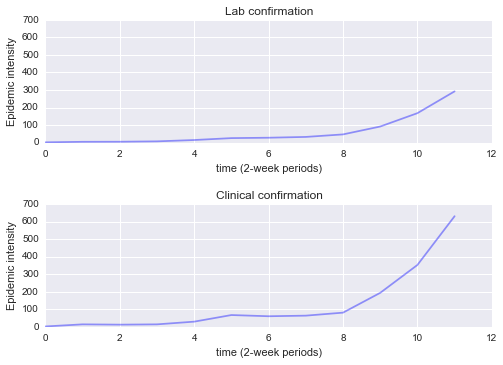In :
S_age_june = pd.DataFrame(model_june.S_age.trace(), columns=age_group.categories).unstack().reset_index()
S_age_june.columns = 'Age', 'Iteration', 'S'
S_age_june['Confirmation'] = 'Lab'

In :
S_age_june = pd.DataFrame(model_june.S_age.trace(),
columns=age_group.categories).unstack().reset_index()
S_age_june.columns = 'Age', 'Iteration', 'S'
S_age_june['Confirmation'] = 'Lab'

S_age_june_noconf = pd.DataFrame(model_june_noconf.S_age.trace(),
columns=age_group.categories).unstack().reset_index()
S_age_june_noconf.columns = 'Age', 'Iteration', 'S'
S_age_june_noconf['Confirmation'] = 'Clinical'

S_age_june = pd.concat([S_age_june, S_age_june_noconf], ignore_index=True)

In :
S_age_july = pd.DataFrame(model_july.S_age.trace(),
columns=age_group.categories).unstack().reset_index()
S_age_july.columns = 'Age', 'Iteration', 'S'
S_age_july['Confirmation'] = 'Lab'

S_age_july_noconf = pd.DataFrame(model_july_noconf.S_age.trace(),
columns=age_group.categories).unstack().reset_index()
S_age_july_noconf.columns = 'Age', 'Iteration', 'S'
S_age_july_noconf['Confirmation'] = 'Clinical'

S_age_july = pd.concat([S_age_july, S_age_july_noconf], ignore_index=True)


Numbers of suscepibles in each age group, under lab vs clinical confirmation

In :
import seaborn as sb
sb.set_context("talk", font_scale=0.8)
sb.set_style("white")

g = sb.factorplot("Age", "S", "Confirmation", S_age_june, kind="box",
palette="hls", size=6, aspect=2, linewidth=0.3, fliersize=0,
order=age_group.categories)
g.despine(offset=10, trim=True)
g.set_axis_labels("Age Group", "Susceptibles");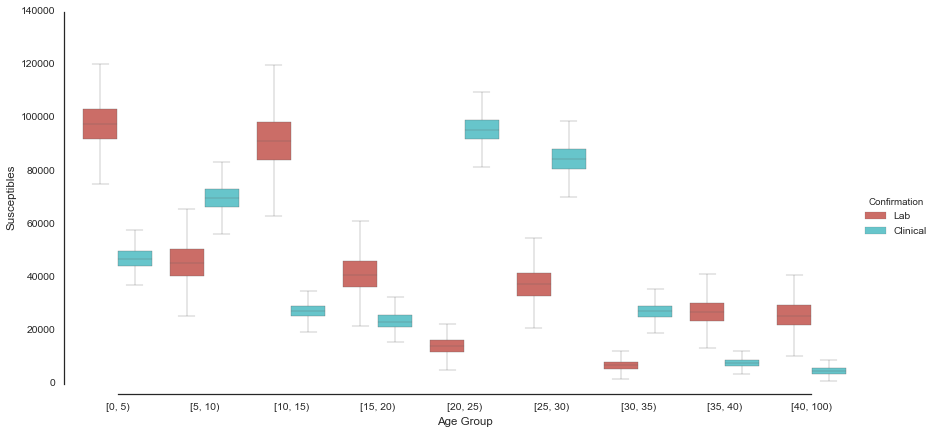In :
june_lam = pd.DataFrame(model_june.λ_t.trace()).unstack().reset_index()
june_lam.columns = ('district', 'iteration', 'λ')
june_lam['month'] = 'June'
june_lam['confirmation'] = 'Lab'

In :
june_lam_noconf = pd.DataFrame(model_june_noconf.λ_t.trace()).unstack().reset_index()
june_lam_noconf.columns = ('district', 'iteration', 'λ')
june_lam_noconf['month'] = 'June'
june_lam_noconf['confirmation'] = 'Clinical'

In :
july_lam = pd.DataFrame(model_july.λ_t.trace()).unstack().reset_index()
july_lam.columns = ('district', 'iteration', 'λ')
july_lam['month'] = 'July'
july_lam['confirmation'] = 'Lab'

In :
july_lam_noconf = pd.DataFrame(model_july_noconf.λ_t.trace()).unstack().reset_index()
july_lam_noconf.columns = ('district', 'iteration', 'λ')
july_lam_noconf['month'] = 'July'
july_lam_noconf['confirmation'] = 'Clinical'

In :
confirmed_lam = june_lam.append(july_lam, ignore_index=True)


Epidemic intensity in June and July (with lab confirmation).

In :
confirmed_lam.groupby('month').boxplot(column='λ', return_type='dict');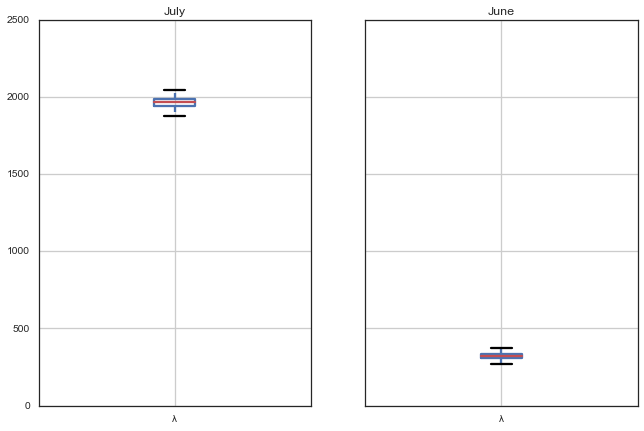Epidemic intensity in June for lab-confirmed and clinical-confirmed.

In :
june_lam = june_lam.append(june_lam_noconf, ignore_index=True)

In :
june_lam.groupby('confirmation').boxplot(column='λ', return_type='dict');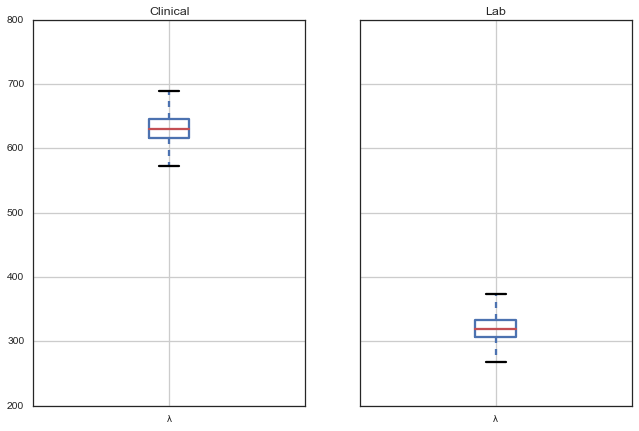In :
model_june.summary(['pct_5', 'pct_15', 'pct_30', 'pct_adult'])

pct_5:

Mean             SD               MC Error        95% HPD interval
------------------------------------------------------------------
0.239            0.021            0.001            [ 0.199  0.281]

Posterior quantiles:

2.5             25              50              75             97.5
|---------------|===============|===============|---------------|
0.2              0.225           0.239          0.253         0.283

pct_15:

Mean             SD               MC Error        95% HPD interval
------------------------------------------------------------------
0.576            0.028            0.002            [ 0.516  0.626]

Posterior quantiles:

2.5             25              50              75             97.5
|---------------|===============|===============|---------------|
0.518            0.555           0.578          0.595         0.63

pct_30:

Mean             SD               MC Error        95% HPD interval
------------------------------------------------------------------
0.803            0.018            0.001            [ 0.767  0.837]

Posterior quantiles:

2.5             25              50              75             97.5
|---------------|===============|===============|---------------|
0.766            0.793           0.804          0.815         0.837

Mean             SD               MC Error        95% HPD interval
------------------------------------------------------------------
0.366            0.027            0.002            [ 0.313  0.416]

Posterior quantiles:

2.5             25              50              75             97.5
|---------------|===============|===============|---------------|
0.315            0.348           0.365          0.383         0.418


In :
june_coverage = pd.DataFrame({name: model_june.trace(name)[:] for name in ['pct_5', 'pct_15', 'pct_30', 'pct_adult']})
june_coverage['Month'] = 'June'
june_coverage['Confirmation'] = 'Lab'

In :
june_noconf_coverage = pd.DataFrame({name: model_june_noconf.trace(name)[:] for name in ['pct_5', 'pct_15', 'pct_30', 'pct_adult']})
june_noconf_coverage['Month'] = 'June'
june_noconf_coverage['Confirmation'] = 'Clinical'

july_coverage = pd.DataFrame({name: model_july.trace(name)[:] for name in ['pct_5', 'pct_15', 'pct_30', 'pct_adult']})
july_coverage['Month'] = 'July'
july_coverage['Confirmation'] = 'Lab'

july_noconf_coverage = pd.DataFrame({name: model_july_noconf.trace(name)[:] for name in ['pct_5', 'pct_15', 'pct_30', 'pct_adult']})
july_noconf_coverage['Month'] = 'July'
july_noconf_coverage['Confirmation'] = 'Clinical'

In :
coverage = pd.concat([june_coverage, june_noconf_coverage, july_coverage, july_noconf_coverage],
ignore_index=True)

In :
sb.factorplot(row="Month", col="Confirmation", data=coverage, kind='box',
row_order=['June', 'July'],
order=['pct_5', 'pct_15', 'pct_30', 'pct_adult'],
palette="YlGnBu_d", linewidth=0.7, fliersize=0, aspect=1.25).despine(left=True)

Out:
<seaborn.axisgrid.FacetGrid at 0x119bce240>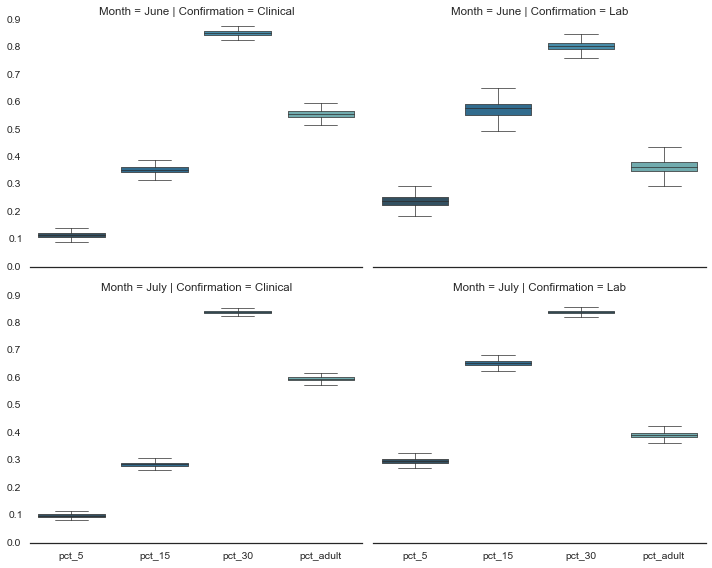In :
Matplot.summary_plot(model_june.p_age)

Could not calculate Gelman-Rubin statistics. Requires multiple chains of equal length.